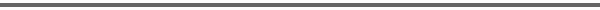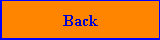Volume 1 V. N. Lagunov Introduction to Differential Games and Control Theory 293 p., soft cover, ISBN 3-88538-401-9, EUR 45.00, 1985 Presents an elementary introduction to the control of discrete and continuous processes and treats in particular the maximum principle and dynamic programming. Then classical n-person games, duels and multi-step games are discussed. Finally the two streams merge into a single channel: the theory of differential games. "This is one of the very few elementary textbooks which deal with both optimal control and differential games ... on the whole, is well written" (Eur. J. Oper. Res.).Volume 2 F. J. Gould, J. W. Tolle Complementary Pivoting on a Pseudomanifold Structure with Applications in the Decision Sciences 207 p., soft cover, ISBN 3-88538-402-7, EUR 10.00, 1983 "... highly recommended to anyone interested in the theory of complementary pivoting techniques. The clear exposition, the exercises at the end of each chapter and the many examples make the text particularly appropriate for classroom use" (Eur. J. Oper. Res.). "The authors succeed in presenting in clear and simple terms a unifying exposition ... the reader gets a good idea how this theory can be applied to solve many highly nonlinear problems arising in the decision sciences" (Optima).Volume 3 K. G. Murty Linear Complementarity, Linear and Nonlinear Programming ISBN 3-88538-403-6, 1988, out of print An electronic version of the book is available freely at: http://ioe.engin.umich.edu/people/fac/books/ murty/linear_complementarity_webbook/Volume 4 B. D. Craven Fractional Programming 150 p., soft cover, ISBN 3-88538-404-3, EUR 25.00, 1988 Discusses the whole range of theoretical and practical questions related to fractional programming. A large number of algorithms are presented to actually solve linear and nonlinear problems. "... introduction to fractional programming ... reaches a depth that makes it attractive also to the researcher ... warmly recommended." (Z. Oper. Res. & Optimization).Volume 5 M. A. Krasnosel'skij, E. A. Lifshits, A. V. Sobolev Positive Linear Systems. The Method of Positive Operators 362 p., soft cover, ISBN 3-88538-405-1, EUR 45.00, 1989 The book is concerned with methods for studying systems and problems whose mathematical description leads to equations involving positive operators with respect to some partial ordering. The first chapter introduces basic notions as partial ordering, various classes of cones and their geometry, ideally convex sets and smoothness points. The second presents applications to spectral problems involving principal eigenvalues and eigendirections, peripheral eigenvalues, focussing and acute operators, and oscillation theorems. The third chapter studies iteration procedures and methods for obtaining estimates for spectral radii and spectral margins, and a posteriori estimates in eigenvector problems. The last chapter is concerned with some applications to forced oscillation problems in automatic control systems, including their construction by means of the harmonic balance method, as well as with stability and absolute stability problems.Volume 6 W. Krabs Mathematical Foundations of Signal Theory 160 p., hard cover, ISBN 3-88538-406-X, EUR 40.00, 1995 The author first discusses discrete, analog, and generalized analog signals, the latter being represented as tempered distributions. In the center of interest are time-invariant, linear communication systems and the question under which conditions the response of an arbitrary input signal can be represented as convolution of the signal with the response of the delta impulse. Further considerations are devoted to the modulation of discrete signals into analog signals and to the question under which conditions exact sampling is possible. Then, Fourier transformations of test functions and distributions are discussed in various spaces of complex-valued measurable functions. The last chapters treat operations with Fourier transformations. In particular it is shown how linear differential equations for distributions with constant coefficients can be solved. Then, Fourier transformations of periodic functions and band-limited signals are described in detail. Shannon's sampling theorem and Poisson's sum formula are proved. After a discussion of discrete Fourier transformation with applications, the book closes with the presentation of fast Fourier transformation, and modulation and sampling of stochastic discrete signals.Volume 7 Rizwan Butt Introduction to Applied Linear Algebra with MATLAB